Share

# Show Variation of Displacement, Velocity and Acceleration with Phase for a Particle Performing Linear S.H.M. Graphically, When It Starts from Extreme Position. - Physics

#### Question

Show variation of displacement, velocity, and acceleration with phase for a particle performing linear S.H.M. graphically, when it starts from the extreme position.

#### Solution

In the equation of S.H.M,
x = A sin (ωt + α)
where, (ωt + α) is the phase or phase angle of S.H.M
For a particle starting from extreme position:
Displacement time graph: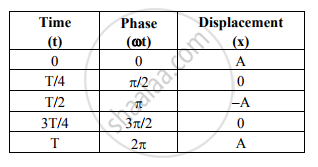Graph: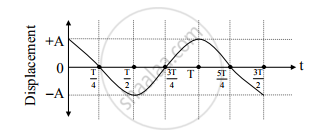Velocity-time graph: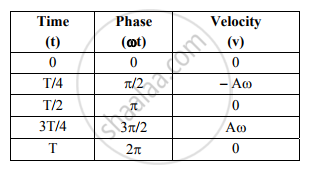Graph: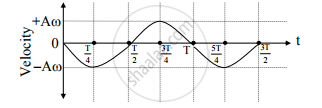Acceleration-time graph: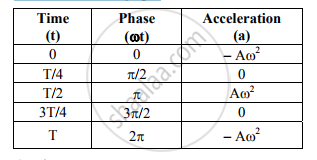Graph: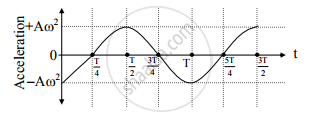Is there an error in this question or solution?